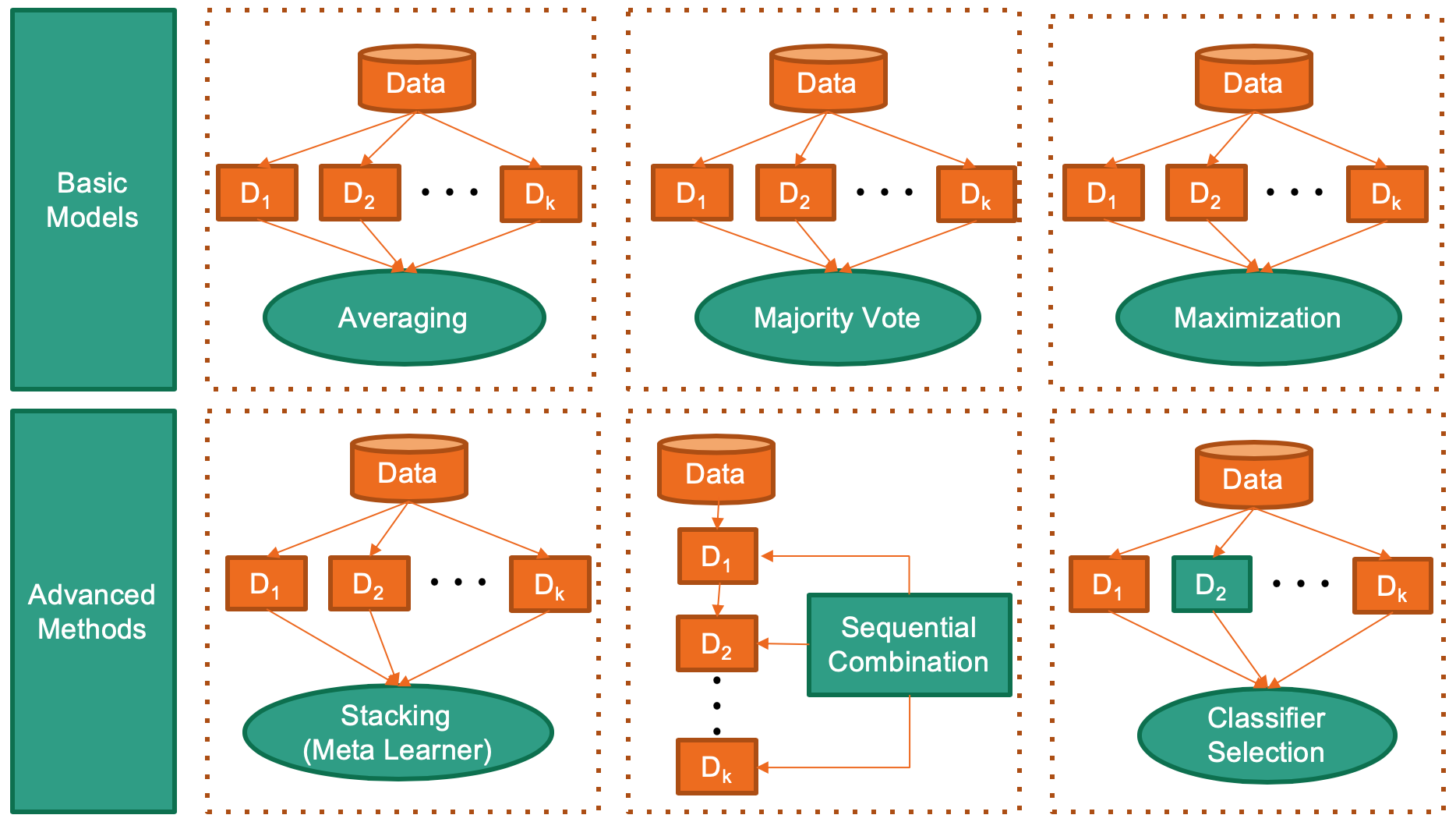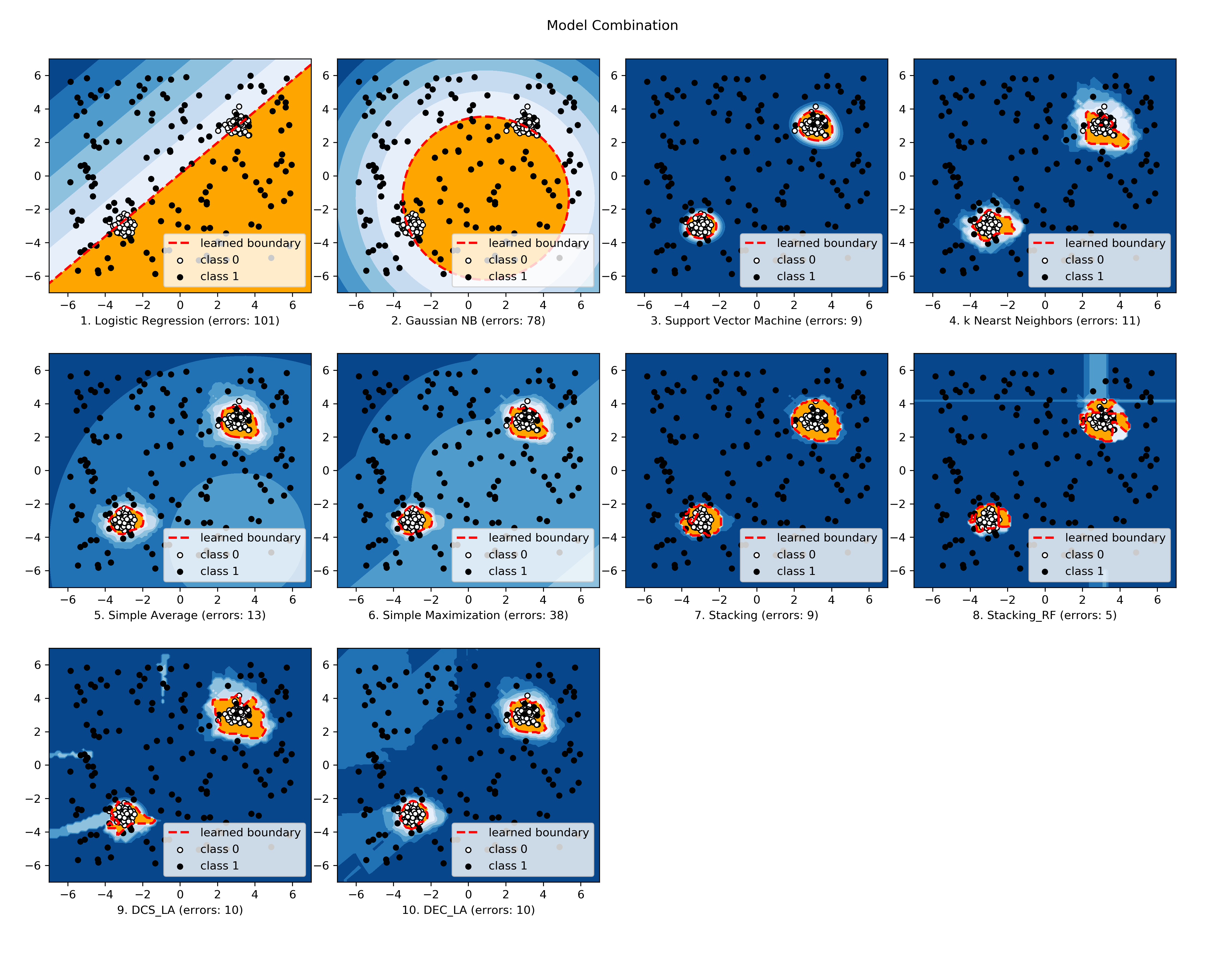Skip to content

# yzhao062/combo

A Python Toolbox for Machine Learning Model Combination
PythonFetching latest commit…
Cannot retrieve the latest commit at this time.
Type Name Latest commit message Commit time
Failed to load latest commit information..circleci Aug 4, 2019combo Aug 8, 2019docs Aug 13, 2019examples Aug 8, 2019notebooks Aug 4, 2019.gitattributes Aug 1, 2019.gitignore Jul 14, 2019.travis.yml Jul 17, 2019CHANGES.txt Aug 8, 2019LICENSE Jul 14, 2019MANIFEST.in Jul 15, 2019README.rst Aug 13, 2019appveyor.yml Jul 28, 2019pypi_build_commands.txt Jul 28, 2019requirements.txt Jul 30, 2019setup.py Aug 6, 2019

# combo: A Python Toolbox for Machine Learning Model Combination

Deployment & Documentation & StatsBuild Status & Coverage & Maintainability & Licensecombo is a comprehensive Python toolbox for combining machine learning (ML) models and scores. Model combination can be considered as a subtask of ensemble learning, and has been widely used in real-world tasks and data science competitions like Kaggle .

combo library supports the combination of models and score from key ML libraries such as scikit-learn and xgboost, and crucial tasks including classification, clustering, anomaly detection. See figure below for some representative combination approaches.combo is featured for:

• Unified APIs, detailed documentation, and interactive examples across various algorithms.
• Advanced and latest models, such as Stacking/DCS/DES/EAC/LSCP.
• Comprehensive coverage for classification, clustering, anomaly detection, and raw score.
• Optimized performance with JIT and parallelization when possible, using numba and joblib.

API Demo:

```from combo.models.classifier_stacking import Stacking
# initialize a group of base classifiers
classifiers = [DecisionTreeClassifier(), LogisticRegression(),
KNeighborsClassifier(), RandomForestClassifier(),
GradientBoostingClassifier()]

clf = Stacking(base_estimators=classifiers) # initialize a Stacking model
clf.fit(X_train, y_train) # fit the model

# predict on unseen data
y_test_labels = clf.predict(X_test)  # label prediction
y_test_proba = clf.predict_proba(X_test)  # probability prediction```

Key Links and Resources:

Table of Contents:

## Installation

It is recommended to use pip for installation. Please make sure the latest version is installed, as combo is updated frequently:

```pip install combo            # normal install
pip install --upgrade combo  # or update if needed
pip install --pre combo      # or include pre-release version for new features```

Alternatively, you could clone and run setup.py file:

```git clone https://github.com/yzhao062/combo.git
cd combo
pip install .```

Required Dependencies:

• Python 3.5, 3.6, or 3.7
• joblib
• matplotlib (optional for running examples)
• numpy>=1.13
• numba>=0.35
• pyod
• scipy>=0.19.1
• scikit_learn>=0.19.1

Note on Python 2: The maintenance of Python 2.7 will be stopped by January 1, 2020 (see official announcement). To be consistent with the Python change and combo's dependent libraries, e.g., scikit-learn, combo only supports Python 3.5+ and we encourage you to use Python 3.5 or newer for the latest functions and bug fixes. More information can be found at Moving to require Python 3.

## API Cheatsheet & Reference

Full API Reference: (https://pycombo.readthedocs.io/en/latest/api.html). The following APIs are consistent for most of the models (API Cheatsheet: https://pycombo.readthedocs.io/en/latest/api_cc.html).

• fit(X, y): Fit estimator. y is optional for unsupervised methods.
• predict(X): Predict on a particular sample once the estimator is fitted.
• predict_proba(X): Predict the probability of a sample belonging to each class once the estimator is fitted.
• fit_predict(X, y): Fit estimator and predict on X. y is optional for unsupervised methods.

For raw score combination (after the score matrix is generated), use individual methods from "score_comb.py" directly. Raw score combination API: (https://pycombo.readthedocs.io/en/latest/api.html#score-combination).

## Implemented Algorithms

combo groups combination frameworks by tasks. General purpose methods are fundamental ones which can be applied to various tasks.

Task Algorithm Year Ref
General Purpose Average & Weighted Average: average across all scores/prediction results, maybe with weights N/A 
General Purpose Maximization: simple combination by taking the maximum scores N/A 
General Purpose Median: take the median value across all scores/prediction results N/A 
General Purpose Majority Vote & Weighted Majority Vote N/A 
Classification SimpleClassifierAggregator: combining classifiers by general purpose methods above N/A N/A
Classification DCS: Dynamic Classifier Selection (Combination of multiple classifiers using local accuracy estimates) 1997 
Classification DES: Dynamic Ensemble Selection (From dynamic classifier selection to dynamic ensemble selection) 2008 
Classification Stacking (meta ensembling): use a meta learner to learn the base classifier results N/A 
Clustering Clusterer Ensemble: combine the results of multiple clustering results by relabeling 2006 
Clustering Combining multiple clusterings using evidence accumulation (EAC) 2002 
Anomaly Detection SimpleDetectorCombination: combining outlier detectors by general purpose methods above N/A 
Anomaly Detection Average of Maximum (AOM): divide base detectors into subgroups to take the maximum, and then average 2015 
Anomaly Detection Maximum of Average (MOA): divide base detectors into subgroups to take the average, and then maximize 2015 
Anomaly Detection XGBOD: a semi-supervised combination framework for outlier detection 2018 
Anomaly Detection Locally Selective Combination (LSCP) 2019 

The comparison among selected implemented models is made available below (Figure, compare_selected_classifiers.py, Interactive Jupyter Notebooks). For Jupyter Notebooks, please navigate to "/notebooks/compare_selected_classifiers.ipynb".All implemented modes are associated with examples, check "combo examples" for more information.

## Example of Stacking/DCS/DES

"examples/classifier_stacking_example.py" demonstrates the basic API of stacking (meta ensembling). "examples/classifier_dcs_la_example.py" demonstrates the basic API of Dynamic Classifier Selection by Local Accuracy. "examples/classifier_des_la_example.py" demonstrates the basic API of Dynamic Ensemble Selection by Local Accuracy.

It is noted the basic API is consistent across all these models.

1. Initialize a group of classifiers as base estimators

```# initialize a group of classifiers
classifiers = [DecisionTreeClassifier(random_state=random_state),
LogisticRegression(random_state=random_state),
KNeighborsClassifier(),
RandomForestClassifier(random_state=random_state),
GradientBoostingClassifier(random_state=random_state)]```
2. Initialize, fit, predict, and evaluate with Stacking

```from combo.models.classifier_stacking import Stacking

clf = Stacking(base_estimators=classifiers, n_folds=4, shuffle_data=False,
keep_original=True, use_proba=False, random_state=random_state)

clf.fit(X_train, y_train)
y_test_predict = clf.predict(X_test)
evaluate_print('Stacking | ', y_test, y_test_predict)```
3. See a sample output of classifier_stacking_example.py

```Decision Tree        | Accuracy:0.9386, ROC:0.9383, F1:0.9521
Logistic Regression  | Accuracy:0.9649, ROC:0.9615, F1:0.973
K Neighbors          | Accuracy:0.9561, ROC:0.9519, F1:0.9662
Gradient Boosting    | Accuracy:0.9605, ROC:0.9524, F1:0.9699
Random Forest        | Accuracy:0.9605, ROC:0.961, F1:0.9693

Stacking             | Accuracy:0.9868, ROC:0.9841, F1:0.9899```

## Example of Classifier Combination

"examples/classifier_comb_example.py" demonstrates the basic API of predicting with multiple classifiers. It is noted that the API across all other algorithms are consistent/similar.

1. Initialize a group of classifiers as base estimators

```# initialize a group of classifiers
classifiers = [DecisionTreeClassifier(random_state=random_state),
LogisticRegression(random_state=random_state),
KNeighborsClassifier(),
RandomForestClassifier(random_state=random_state),
GradientBoostingClassifier(random_state=random_state)]```
2. Initialize, fit, predict, and evaluate with a simple aggregator (average)

```from combo.models.classifier_comb import SimpleClassifierAggregator

clf = SimpleClassifierAggregator(classifiers, method='average')
clf.fit(X_train, y_train)
y_test_predicted = clf.predict(X_test)
evaluate_print('Combination by avg   |', y_test, y_test_predicted)```
3. See a sample output of classifier_comb_example.py

```Decision Tree        | Accuracy:0.9386, ROC:0.9383, F1:0.9521
Logistic Regression  | Accuracy:0.9649, ROC:0.9615, F1:0.973
K Neighbors          | Accuracy:0.9561, ROC:0.9519, F1:0.9662
Gradient Boosting    | Accuracy:0.9605, ROC:0.9524, F1:0.9699
Random Forest        | Accuracy:0.9605, ROC:0.961, F1:0.9693

Combination by avg   | Accuracy:0.9693, ROC:0.9677, F1:0.9763
Combination by w_avg | Accuracy:0.9781, ROC:0.9716, F1:0.9833
Combination by max   | Accuracy:0.9518, ROC:0.9312, F1:0.9642
Combination by w_vote| Accuracy:0.9649, ROC:0.9644, F1:0.9728
Combination by median| Accuracy:0.9693, ROC:0.9677, F1:0.9763```

## Example of Clustering Combination

"examples/cluster_comb_example.py" demonstrates the basic API of combining multiple base clustering estimators. "examples/cluster_eac_example.py" demonstrates the basic API of Combining multiple clusterings using evidence accumulation (EAC).

1. Initialize a group of clustering methods as base estimators

```# Initialize a set of estimators
estimators = [KMeans(n_clusters=n_clusters),
MiniBatchKMeans(n_clusters=n_clusters),
AgglomerativeClustering(n_clusters=n_clusters)]```
2. Initialize a Clusterer Ensemble class and fit the model

```from combo.models.cluster_comb import ClustererEnsemble
# combine by Clusterer Ensemble
clf = ClustererEnsemble(estimators, n_clusters=n_clusters)
clf.fit(X)```
3. Get the aligned results

```# generate the labels on X
aligned_labels = clf.aligned_labels_
predicted_labels = clf.labels_```

## Example of Outlier Detector Combination

"examples/detector_comb_example.py" demonstrates the basic API of combining multiple base outlier detectors.

1. Initialize a group of outlier detection methods as base estimators

```# Initialize a set of estimators
detectors = [KNN(), LOF(), OCSVM()]```
2. Initialize a simple averaging aggregator, fit the model, and make the prediction.

```from combo.models.detector combination import SimpleDetectorAggregator
clf = SimpleDetectorAggregator(base_estimators=detectors)
clf_name = 'Aggregation by Averaging'
clf.fit(X_train)

y_train_pred = clf.labels_  # binary labels (0: inliers, 1: outliers)
y_train_scores = clf.decision_scores_  # raw outlier scores

# get the prediction on the test data
y_test_pred = clf.predict(X_test)  # outlier labels (0 or 1)
y_test_scores = clf.decision_function(X_test)  # outlier scores```
3. Evaluate the prediction using ROC and Precision @ Rank n.

```# evaluate and print the results
print("\nOn Training Data:")
evaluate_print(clf_name, y_train, y_train_scores)
print("\nOn Test Data:")
evaluate_print(clf_name, y_test, y_test_scores)```
4. See sample outputs on both training and test data.

```On Training Data:
Aggregation by Averaging ROC:0.9994, precision @ rank n:0.95

On Test Data:
Aggregation by Averaging ROC:1.0, precision @ rank n:1.0```

## Development Status

combo is currently under development as of July 30, 2019. A concrete plan has been laid out and will be implemented in the next few months.

Similar to other libraries built by us, e.g., Python Outlier Detection Toolbox (pyod), combo is also targeted to be published in Journal of Machine Learning Research (JMLR), open-source software track. A demo paper to AAAI or IJCAI may be submitted soon for progress update.

Watch & Star to get the latest update! Also feel free to send me an email (zhaoy@cmu.edu) for suggestions and ideas.

## Inclusion Criteria

Similarly to scikit-learn, We mainly consider well-established algorithms for inclusion. A rule of thumb is at least two years since publication, 50+ citations, and usefulness.

However, we encourage the author(s) of newly proposed models to share and add your implementation into combo for boosting ML accessibility and reproducibility. This exception only applies if you could commit to the maintenance of your model for at least two year period.

## Reference

  (1, 2) Aggarwal, C.C. and Sathe, S., 2015. Theoretical foundations and algorithms for outlier ensembles. ACM SIGKDD Explorations Newsletter, 17(1), pp.24-47.
  Aggarwal, C.C. and Sathe, S., 2017. Outlier ensembles: An introduction. Springer.
  Bell, R.M. and Koren, Y., 2007. Lessons from the Netflix prize challenge. SIGKDD Explorations, 9(2), pp.75-79.
  Gorman, B. (2016). A Kaggler's Guide to Model Stacking in Practice. [online] The Official Blog of Kaggle.com. Available at: http://blog.kaggle.com/2016/12/27/a-kagglers-guide-to-model-stacking-in-practice [Accessed 26 Jul. 2019].
  Ko, A.H., Sabourin, R. and Britto Jr, A.S., 2008. From dynamic classifier selection to dynamic ensemble selection. Pattern recognition, 41(5), pp.1718-1731.
  Fred, A. L. N., & Jain, A. K. (2005). Combining multiple clusterings using evidence accumulation. IEEE Transactions on Pattern Analysis and Machine Intelligence, 27(6), 835–850. https://doi.org/10.1109/TPAMI.2005.113
  Woods, K., Kegelmeyer, W.P. and Bowyer, K., 1997. Combination of multiple classifiers using local accuracy estimates. IEEE transactions on pattern analysis and machine intelligence, 19(4), pp.405-410.
  Zhao, Y., Nasrullah, Z., Hryniewicki, M.K. and Li, Z., 2019, May. LSCP: Locally selective combination in parallel outlier ensembles. In Proceedings of the 2019 SIAM International Conference on Data Mining (SDM), pp. 585-593. Society for Industrial and Applied Mathematics.
  Zhao, Y. and Hryniewicki, M.K. XGBOD: Improving Supervised Outlier Detection with Unsupervised Representation Learning. IEEE International Joint Conference on Neural Networks, 2018.
  Zhou, Z.H. and Tang, W., 2006. Clusterer ensemble. Knowledge-Based Systems, 19(1), pp.77-83.
  (1, 2, 3, 4) Zhou, Z.H., 2012. Ensemble methods: foundations and algorithms. Chapman and Hall/CRC.
You can’t perform that action at this time.